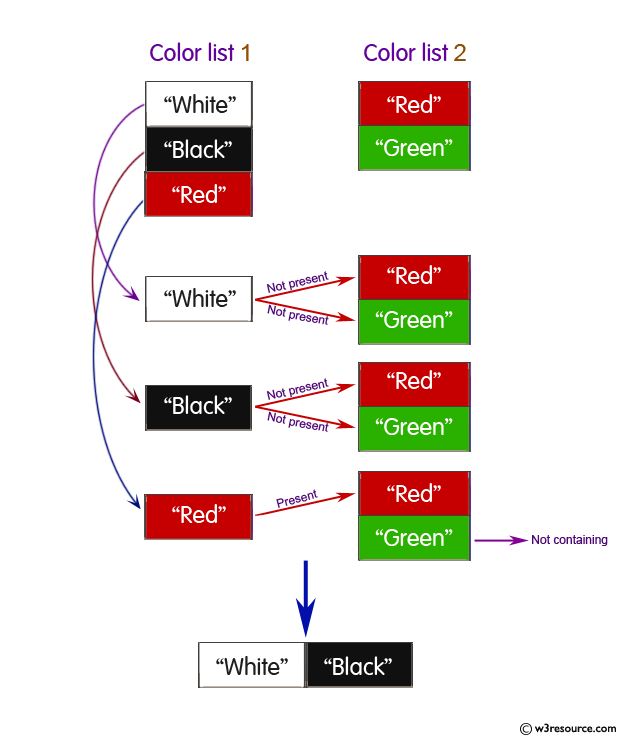﻿ Python: Print out a set containing all the colors from a list which are not present in other list - w3resource# Python: Print out a set containing all the colors from a list which are not present in other list

## Python Basic: Exercise-29 with Solution

Write a Python program to print out a set containing all the colors from color_list_1 which are not present in color_list_2.

Test Data:
color_list_1 = set(["White", "Black", "Red"])
color_list_2 = set(["Red", "Green"])
Expected Output :
{'Black', 'White'}

Pictorial Presentation:Sample Solution-1:

Python Code:

``````color_list_1 = set(["White", "Black", "Red"])
color_list_2 = set(["Red", "Green"])
print("Original set elements:")
print(color_list_1)
print(color_list_2)
print("\nDifferenct of color_list_1 and color_list_2:")
print(color_list_1.difference(color_list_2))
print("\nDifferenct of color_list_2 and color_list_1:")
print(color_list_2.difference(color_list_1))
```
```

Sample Output:

```Original set elements:
{'White', 'Black', 'Red'}
{'Green', 'Red'}

Differenct of color_list_1 and color_list_2:
{'White', 'Black'}

Differenct of color_list_2 and color_list_1:
{'Green'}
```

## Visualize Python code execution:

The following tool visualize what the computer is doing step-by-step as it executes the said program:

Sample Solution-2:

Python Code:

``````color_list_1 = set(["White", "Black", "Red"])
color_list_2 = set(["Red", "Green"])
print("Original set elements:")
print(color_list_1)
print(color_list_2)
print("\nDifferenct of color_list_1 and color_list_2:")
print(color_list_1 - color_list_2)
print("\nDifferenct of color_list_2 and color_list_1:")
print(color_list_2 - color_list_1)
```
```

Sample Output:

```Original set elements:
{'Red', 'Black', 'White'}
{'Red', 'Green'}

Differenct of color_list_1 and color_list_2:
{'Black', 'White'}

Differenct of color_list_2 and color_list_1:
{'Green'}
```

## Visualize Python code execution:

The following tool visualize what the computer is doing step-by-step as it executes the said program:

Python Code Editor:

Have another way to solve this solution? Contribute your code (and comments) through Disqus.

What is the difficulty level of this exercise?

Test your Python skills with w3resource's quiz

﻿

## Python: Tips of the Day

Try-catch-else construct:

```try:
foo()
except Exception:
print("Exception occured")
else:
print("Exception didnt occur")
finally:
print("Always gets here")
```